# Namioka theorem

(diff) ← Older revision | Latest revision (diff) | Newer revision → (diff)

Letbe a regular, strongly countably complete topological space (cf. also Strongly countably complete topological space), letbe a locally compact and-compact space (cf. also Compact space) and let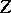be a pseudo-metric space. In 1974, I. Namioka [a7] proved that for every separately continuous functionthere is a dense-subsetofsuch that the set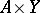is contained in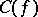, the set of points of continuity of(cf. also Set of type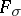(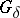); Separate and joint continuity).

The original proof of this theorem starts with an interesting reduction to the case whenis compact. Next, using purely topological methods, such as, e.g., the Arkhangel'skii–Frolík covering theorem and Kuratowski's theorem on closed projections, Namioka shows that, given that the set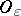is the union of all open subsetsof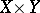such that, the setis dense in.

For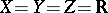(the real numbers), such a result was known already to R. Baire [a2] (cf. Separate and joint continuity).

Ifis complete metric,is compact metric and, Namioka's theorem was shown by H. Hahn [a6] (see also [a11]).

The question whether the completeness ofsuffices in Hahn's result was asked, independently, in [a1] and [a5]. The following example, due to J.B. Brown [a8] shows that completeness does not suffice and proves the necessity of compactness of. In fact, let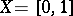,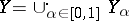, where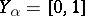anddenotes the free union of, in fact,many copies of. Let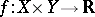be separately continuous on every "square"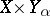and having a point of discontinuity along the line. Then, clearly, the setmentioned in Namioka's theorem is empty.

Answering a problem of Namioka, it was shown [a12] that Namioka's theorem fails for all Baire spaces(cf. also Baire space). Still, the theorem holds for certain Banach–Mazur game-defined spaces (cf. also Banach–Mazur game), namely for--defavourable spaces [a3], [a10] and for Baire spaces having dense subsets that are countable unions of-analytic subsets [a13].

The importance of Namioka's theorem lies in the fact that bothandare neither metrizable nor having any kind of countability of basis.

Ifhas a countable base, then Namioka's theorem holds for all Baire spaces, see [a4] and [a9].

For further information, see Namioka space.

How to Cite This Entry:
Namioka theorem. Encyclopedia of Mathematics. URL: http://encyclopediaofmath.org/index.php?title=Namioka_theorem&oldid=11989
This article was adapted from an original article by Z. Piotrowski (originator), which appeared in Encyclopedia of Mathematics - ISBN 1402006098. See original article